# Auto Control 002 自动控制原理 自动控制的数学模型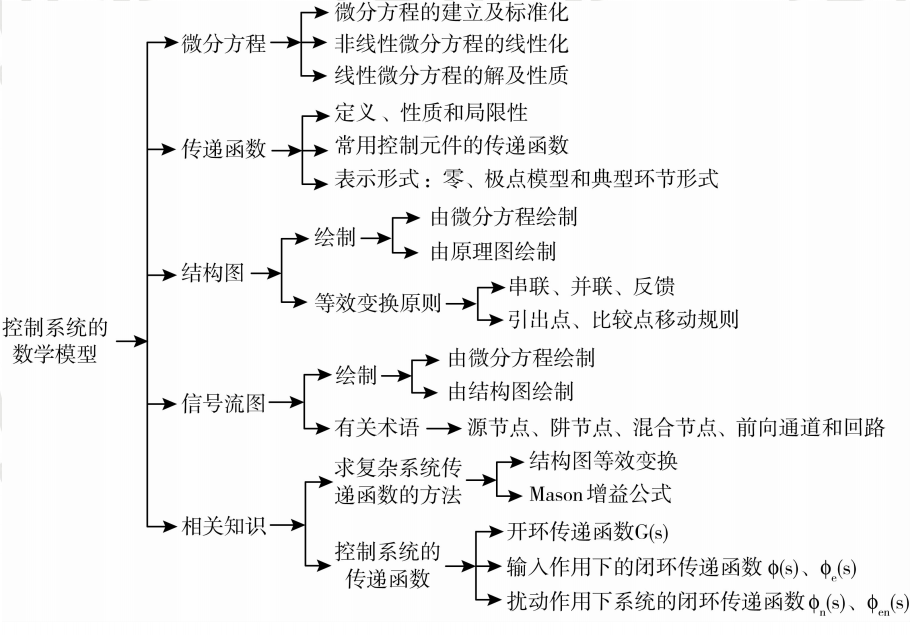## 一 . 微分方程

1 . 微分方程它的建立以及如何化成标准化（什么叫做标准化？所谓标准化是指：我们把微分方程的左右分布安装输出和输入的 各阶导数，以及导数从高到低的次序来排列）。

2 . 此外，微分方程中，除了线性系统之外，还有一些非线性系统的微分方程。（不解释）

3 . 列写这些微分方程的目的是什么呢？我们要通过这些微分方程的解来分析系统的性能。这是我们在时域当中要见到的一种数学模型，这种数学模型（微分方程）它的列写是有一定的技巧的，也就是说我们拿到一些系统以后我们要来分析这些系统它的工作性质，究竟是什么样的系统？是力学系统呢，还是热学系统，还是电学系统。按照这些系统它所依据的物理定律，比如说 ：物理学系统它说依据的是牛顿第二定律，而电学系统主要依据的是基尔霍夫电压或者电流定律，列出来原始平衡方程，对这些原始平衡方程进行化解得到标准形式，并且利用微分方程的求解得到微分方程的解，从这些解当中，我们分析控制系统的性能。

## 二 . 传递函数

1 . 那么对于这样的数学模型，我们要了解它的定义，以及它的一些性质。尤其是知道了它的性质，在我们分析系统的响应的时候也会起到简化的作用；还有传递函数在描述系统的时候是不是还有一些局限性，这我们也需要了解。

2 . 还需要了解一些常用的控制元件它的数学模型，也就是它的传递函数。

（1）. 一个是以零、极点的模型形式来表现的。我们可以把它写作：一个系统的传递函数 等于 所对应的所有的系统的零点它的乘积 比上 所有的极点它的乘积。这种数学模型在我们后面介绍根轨迹方法当中，经常会见到。

$$G(s) = \frac{K\prod{m}^{i=1}(s - z{i})}{\prod{n}^{j=1}(s - p{j})}$$

（2） . 除了零、极点这种数学模型之外，还有一种数学模型是以典型环节的形式来表述的，也就是说 这个系统的数学模型，我们能够把它写做：传递函数 等于 由一些比例环节或者是一阶的微分环节或者是二阶的微分环节 比上 积分环节或者一阶惯性环节或者二阶的震荡环节等等，这样的形式来表示的，这样的数学模型的形式，我们在频域分析法当中经常会见到。

## 三 . 动态结构图

（在各个院校的考研试卷中，动态结构图的题即使不是一专门的题来出现，也会在各种题型中出现，因为从系统的动态结构图建立它的传递函数这一过程，是分析系统的基础，所以这种动态结构图的等效我们也需要牢牢的掌握。）

## 四 . 信号流图

• 如果一个时域当时的微分方程或者代数方程我们已经知道了，我们就可以从方程当中来绘制它的信号流图。
• 如果动态结构图我们已经知道了，那么我们从动态结构图中，也能绘制信号流图。

• 传递函数该如何求取
• 动态结构图的化简
• Mason增益公式的使用。

## 五 . 传递函数的定义

$$G(s) = \frac {C(s)}{R(s)}$$

$$a{0} \frac{d^{n}}{dt^{n}}c(t) + a{1} \frac{d^{n-1}}{dt^{n-1}}c(t) + \cdots + a{n-1} \frac{d}{dt}c(t) + a{n}c(t) = b{0}\frac{d^{m}}{dt^{m}}r(t) + b{1}\frac{d^{m-1}}{dt^{m-1}}r(t) + \cdots + b{m-1}\frac{d}{dt}r(t) + b{m}r(t)$$

$$a{0}s^{n}C(s) + a{1} s^{n-1}C(s) + \cdots + a{n-1} sC(s) + a{n}C(s) = b{0}s^{m}R(s) + b{1}s^{m-1}R(s) + \cdots + b{m-1}sR(s) + b{m}R(s)$$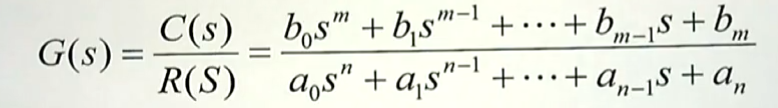## 六 . 传递函数的性质

1 . 由于现实世界当中能量的传递总是有限的，所以微分方程它的输出端的阶次总是会高于输入端的阶次，那么所对应的传递函数它的分子多项式的阶次就一定会低于（最多能过达到等于）分母多项式的最高阶次，并且我们的传递函数描述的是一个线性定常的系统 ，所以所对应的所有系数都是实数 。

2 . 传递函数描述的是系统输入输出之间的关系式，这种关系式它只取决于系统的绝对参数，而和系统的输入信号是什么是没有关系的。也就是说传递函数是系统的一种固有属性，它和外加的激励是没有关系的，当然和系统的初始条件也没有关系，这在传递函数的定义当中我们就可以了解到，传递函数我们是在零初始条件下来定义的。

3 . 传递函数和微分方程之间是可以相互转换的，因此也是一一对应的，这里也不需要多说。

4 . （做题的时候可能会见到） 传递函数的拉氏反变换是系统的单位脉冲响应。 为什么会是这样呢？传递函数我们是这样定义的：$G(s) = \frac {C(s)}{R(s)}$（输出的拉氏变换与输入的拉氏变换在零初始条件下所对应的比值）

5 . 传递函数只是对系统的数学描述，并不反应系统的物理构成。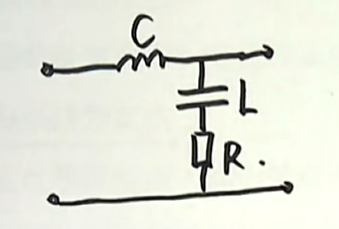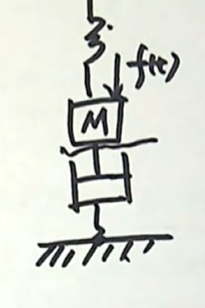$$a{0}\frac {d^{2}c(t)}{dt^{2}} + a{1}\frac {dc(t)}{dt} + a_{2}c(t)$$

## 七 . 那么我们如何来建立系统的数学模型呢？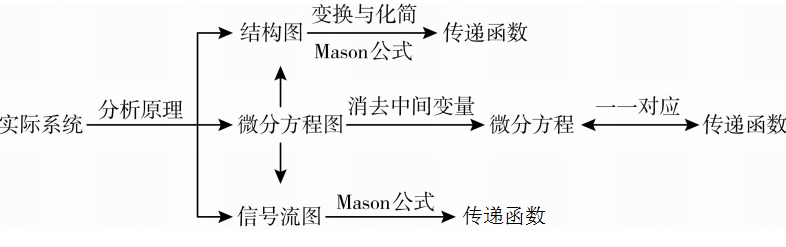## 八 . 对于控制类的考生来说最为常见的的控制系统是这样几个：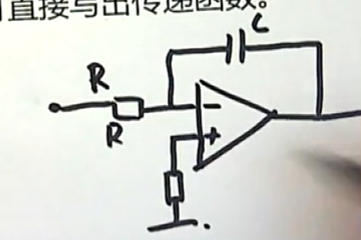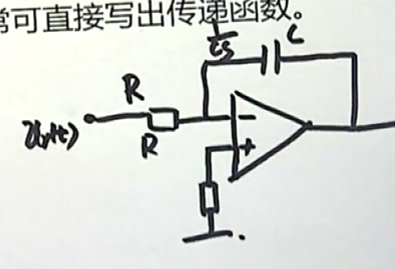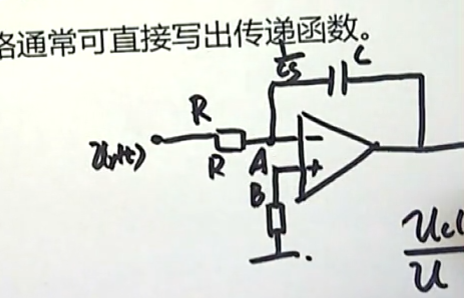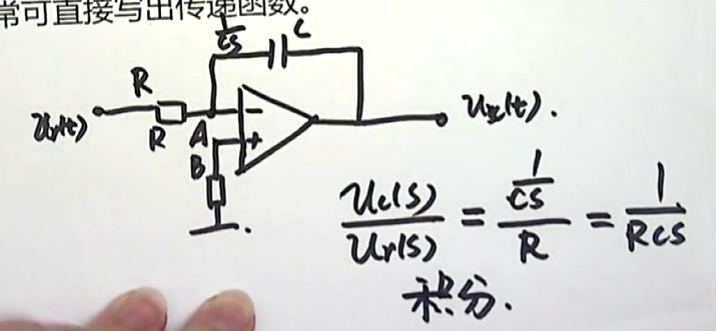## （1）动态结构图的绘制：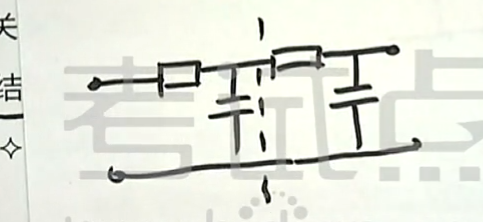## 十 . 动态结构图的变换与化简

1 . 比较点前移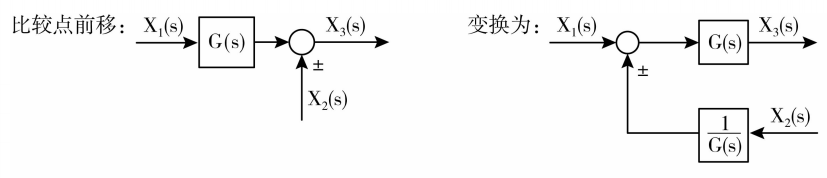$$X{3}(s) = X{1}(s)\cdot G(s) \pm X_{2}(s)$$

$$X{3}(s) = [X{1}(s)\pm X{2}(s)\cdot \frac {1}{G(s)}] \cdot G(s)$$ $$= X{1}(s)\cdot G(s) \pm X_{2}(s)$$

2 . 比较点后移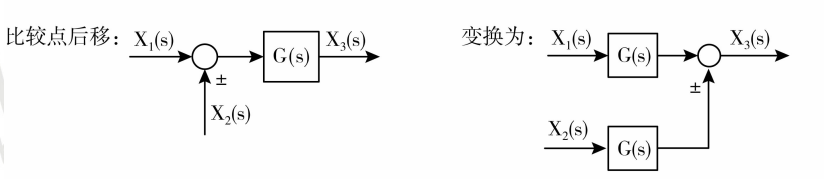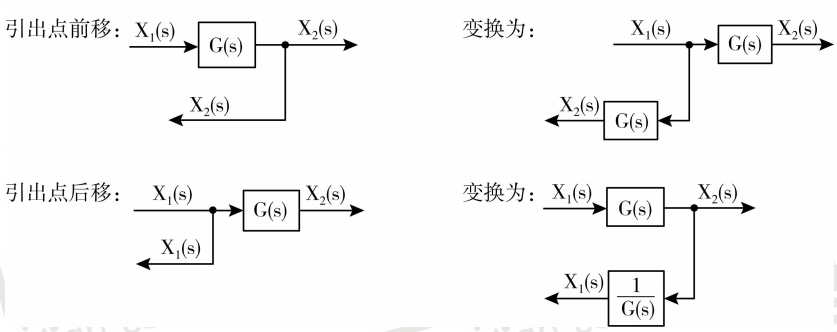（现在先不讲 Mason公式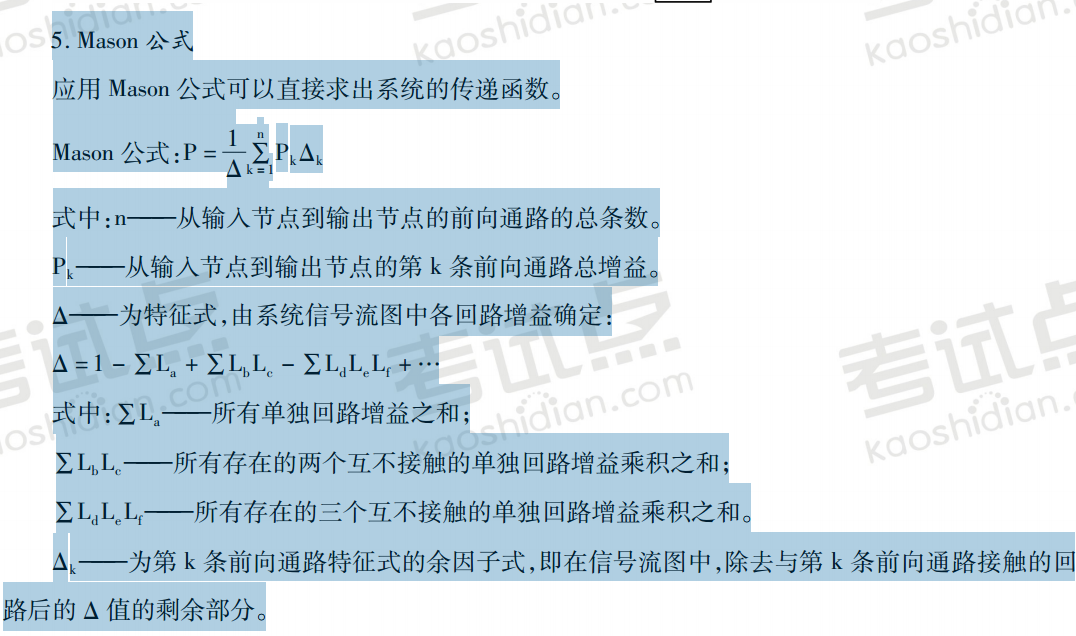## 十一 . 考研要点

1 . 如何建立控制系统的微分方程（有源网络和无源网络如何列写它的微分方程）

2 . 传递函数的概念、性质以及表示形式（表示形式有两种：零、极点的表示形式（跟轨迹增益）； 典型环节相互串联的形式（系统增益））

3 . 动态结构图的等效变换

4 . 求复杂系统的传递函数